# Colpitts Oscillator: What is it? (Circuit Diagram & How To Calculate Frequency of colpitts oscillator)

Contents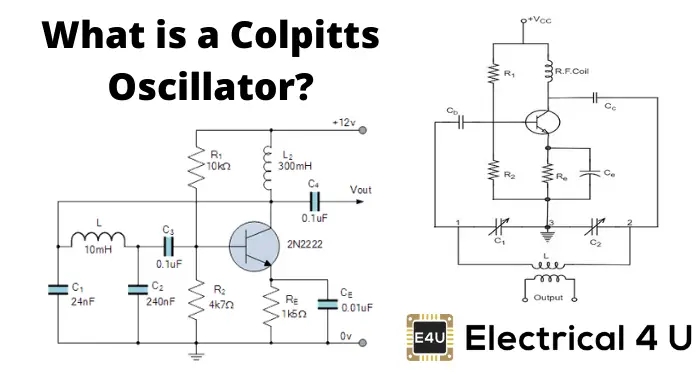## What is a Colpitts Oscillator?

A Colpitts Oscillator is a type of LC oscillator. Colpitts oscillators were invented by American engineer Edwin H. Colpitts in 1918. Like other LC oscillators, Colpitts oscillators use a combination of inductors (L) and capacitors (C) to produce an oscillation at a certain frequency. The distinguishing feature of the Colpitts oscillator is that the feedback for the active device is taken from a voltage divider made of two capacitors in series across the inductor.

That sounds… kind of confusing.

So let’s look at a Colpitts oscillator circuit to understand just how this works.

## Colpitts Oscillator Circuit

Figure 1 shows a typical Colpitts oscillator with a tank circuit. An inductor L is connected parallel to the serial combination of capacitors C1 and C2 (shown by the red enclosure).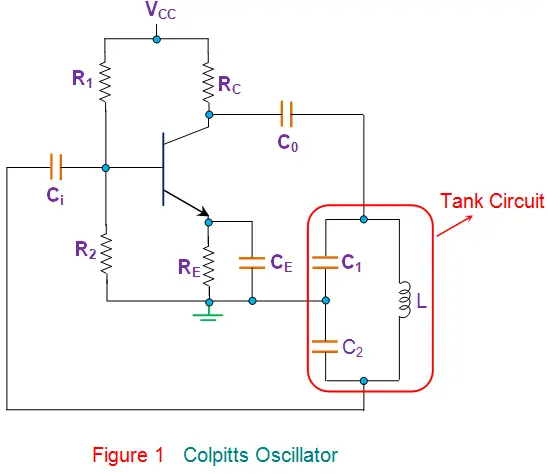Other components in the circuit are the same as that found in the case of common-emitter CE, which is biased using a voltage divider network, i.e., RC is the collector resistor, RE is the emitter resistor which is used to stabilize the circuit, and the resistors R1 and R2 form the voltage divider bias network.

Further, the capacitors Ci and Co are the input and output decoupling capacitors while the emitter capacitor CE is the bypass capacitor used to bypass the amplified AC signals.

Here, as the power supply is switched ON, the transistor starts to conduct, increasing the collector current IC due to which the capacitors C1 and C2 get charged. On acquiring the maximum charge feasible, they start to discharge via the inductor L.

During this process, the electrostatic energy stored in the capacitor gets converted into magnetic flux, which is stored within the inductor in the form of electromagnetic energy.

Next, the inductor starts to discharge, which charges the capacitors once again. Likewise, the cycle continues, which gives rise to the oscillations in the tank circuit.

Further the figure shows that the output of the amplifier appears across C1 and thus is in-phase with the tank circuit’s voltage and makes-up for the energy lost by re-supplying it.

On the other hand, the voltage feedback to the transistor is obtained across the capacitor C2, which means the feedback signal is out-of-phase with the voltage at the transistor by 180o.

This is due to the fact that the voltages developed across the capacitors C1 and C2 are opposite in polarity as the point where they join is grounded.

Further, this signal is provided with an additional phase-shift of 180o by the transistor which results in a net phase-shift of 360o around the loop, satisfying the phase-shift criterion of Barkhausen principle.

## Colpitts Oscillator Frequency

At this stage, the circuit can effectively act as an oscillator producing sustained oscillations by carefully monitoring the feedback ratio given by (C1 / C2). The frequency of such a Colpitts Oscillator depends on the components in its tank circuit and is given by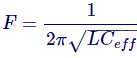Where the Ceff is the effective capacitance of the capacitors expressed as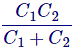As a result, these oscillators can be tuned either by varying their inductance or the capacitance. However, the variation of L does not yield a smooth variation.

Hence they are usually tuned by varying the capacitances which are generally ganged, due to which a change in any one of them changes both of them. Nevertheless, the process is tedious and requires a special large-valued capacitor.

Thus, the Colpitts oscillators are seldom preferred in applications wherein the frequency varies but are more popular as fixed frequency oscillators due to their simple design.

Further, they offer better stability than the Hartley Oscillators as they are exempted from the mutual inductance effect present in-between the two inductors of the latter case.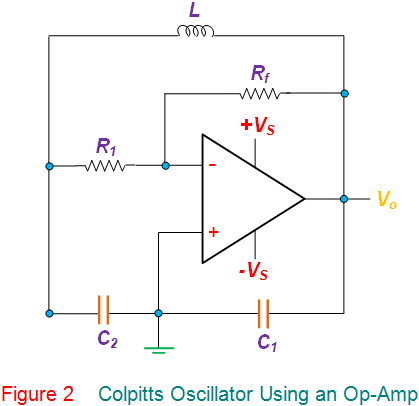Apart from the BJT-based Colpitts Oscillator shown, they are also realizable using valves or FET (Field Effect Transistor) or Op-Amp.

Figure 2 shows such a Colpitts oscillator which uses an Op-Amp in inverting configuration in its amplifier section. At the same time, the tank circuit remains similar to that in the case of Figure 1.

This kind of circuit functions almost analogous to that of the one explained earlier. However, here the gain of the oscillator can be adjusted individually just by using the feedback resistor Rf, as the gain of the inverting amplifier is given as -Rf / R1.

From this, it can be noted that, in this case, the gain of the circuit is less dependent on the circuit elements of the tank circuit.

Typically, the operating frequency of the Colpitts oscillators ranges from 20 kHz to 300 MHz. However, they can even be used for microwave applications as their capacitors provide a low reactance path for high-frequency signals.

This results in better frequency stability as well as a better sinusoidal output waveform. Moreover, they are also extensively used as surface acoustical wave (SAW) resonators, sensors and in mobile and communication systems.

Want To Learn Faster? 🎓
Get electrical articles delivered to your inbox every week.
No credit card required—it’s 100% free.